# Xiaojun Liu

•### Differential Geometry

Book

Xiaojun Liu

•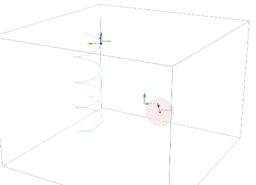### The curvature and Frenet trihedron for spiral curve

Activity

Xiaojun Liu

•### The tangent and curvature of circle

Activity

Xiaojun Liu

•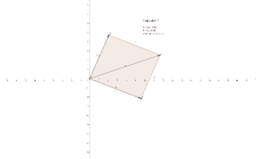### Visual Complex Analysis

Book

Xiaojun Liu

•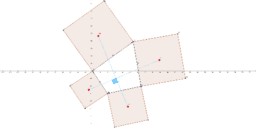### Prove a quadrilateral relation by using complex analysis.

Activity

Xiaojun Liu

•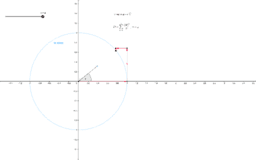### Demonstrate the power series way of proving Euler formula

Activity

Xiaojun Liu

•### Demonstrates the Euler's formula by using motion of particle

Activity

Xiaojun Liu

•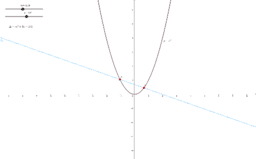### quadratic equation [math] x^2=mx+n [/math]

Activity

Xiaojun Liu

•### Solving the cubic equation [math]x^3 = 3px +2q[/math]

Activity

Xiaojun Liu

•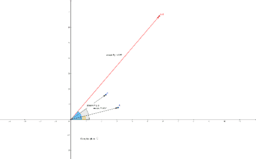### The rules for multiplication of two complex numbers, A B

Activity

Xiaojun Liu

•Activity

Xiaojun Liu